# A teaser question: Can you prove this inequality?

Recently I am working on part 20 to the basics of the 4D complex numbers. Ok ok if you need 20 parts to explain ‘the basics’ how basic is it you can ask yourself.

You can argue long and short on this: are fresh Cauchy integral formula’s really ‘basic stuff’? I don’t know how a democratic vote among professional math professors would fall down.

Anyway, an important property of the determinant of 4D complex numbers is the fact that the determinant is always non-negavite. At least it is zero and at those points in space we have found a non-invertible number.

In part 20 on the basics to 4D complex numbers we will look when the eigenvalues of 4D complex numbers vanish; at those points the stuff is non-invertible & that is what we will be hunting on part 20.

In the next picture you see a difficult to understand inequality & the teaser question is:
Can you prove this inequality via math methods that do not use 4D complex number theory at all?

If so, you should definitely pop up a second pint of perfect beer on a late Friday evening.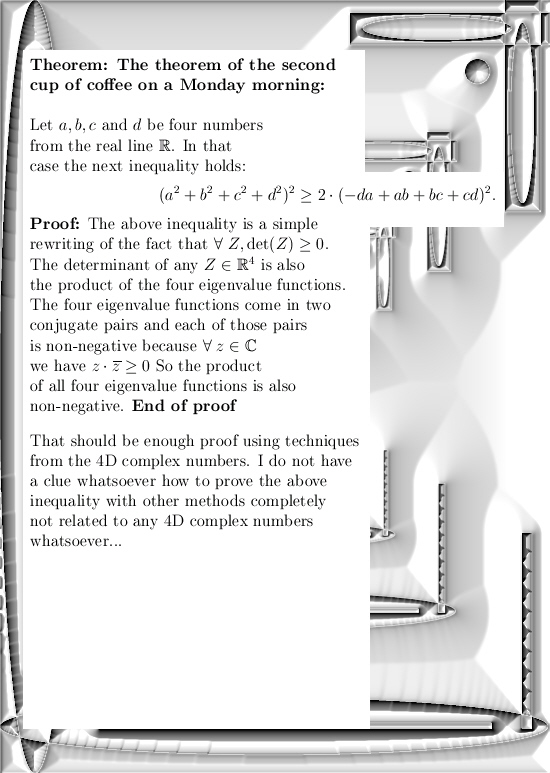Ok, that was it. Till updates in part 20 where we try to find all non-invertible 4D complex numbers in a not too difficult way.

# The inverse theorem of Pythagoras (part 2).

Somewhere last year I just looked some nice video from the Mathologer about the theorem of Pythagoras. And since I myself have found a proof for the general theorem of Pythagoras in higher dimensions, I was puzzled about what the so called ‘inverse theorem of Pythagoras’ actually was.

Could I do that too in my general proof? And the answer was yes, but when I wrote that old proof of the general theorem of Pythagoras it was just a technical blip not worthwhile mentioning because it was a simple consequence of how those normal vectors work.

Anyway to make a long story short, a few days back I likely had nothing better to do and for some reason I did an internet search for ‘the inverse theorem of Pythagoras’. All I wanted to do is read a bit more about that from other people.

To my surprise my own writing popped up as search result number 3, that was weird because I wanted to read stuff written by other people… Here is a screenshot of the answers as given by the Google search machine:Ok ok, not bad at search result number 3.

Now why bring this up? Well originally I forgot to post to the video that started my thinking in the first place. It is from the Mathologer and here at 16.00 minutes into his video is where my mind started to drift off:The video from the Mathologer is here (title Visualizing Pythagoras: ultimate proofs and crazy contortions):

It is a very good video, my compliments.

After so much advertisements for the Mathologer, just a tiny advertisement for what I wrote on the subject of the inverse theorem of Pythagoras on March 20 in the year 2018:

What is the inverse Pythagoras theorem?

Ok, that was it. Till updates.

# Part 19: Four integrals defining the 4D complex number tau.

It is a bit late but a happy new year anyway! In this post we will do a classic from the complex plane: calculation of the log of the first imaginary unit.

On the complex plane this is log i and on the complex 4D space this is log l .

Because this number is so important I have given it a separate name a long long time ago: These are the numbers tau in the diverse dimensions. In the complex plane it has no special name and it simply is i times pi/2.

On the real line it is pretty standard to define the log functions as the integral of the inverse 1/x. After all the derivative of log x on the real line is 1/x and as such you simply define the log to be the integral of the derivative…

On the complex plane you can do the same but depending of how your path goes around zero you can get different answers. Also in the complex plane (and other higher dimensional number systems) the log is ‘multi valued’. That is a reflection of the fact we can find exponential periodic functions also known as the exponential circles and curves.

The integrals in this part number 19 on the basics of 4D complex numbers are very hard to crack. I know of no way to find primitives and to crack them that way. May be that is possible, may be it is not, I just do not know. But because I developed the method of matrix diagonals for finding expressions for the value of those difficult looking integrals, more or less in an implicit manner we give the right valuations to those four integrals.

With the word ‘implicit’ I simply mean we skip the whole thing of caculating the number tau via matrix diagonalization. We only calculate what those integrals actually are in terms of a half circle with coordinates cos t and sin t.

This post is 8 pictures long in the usual size of 550 by 775 pixels (I had to enlarge the latest picture a little bit). I hope it is not loaded with typo’s any more and you have a more or less clean mathematical experience: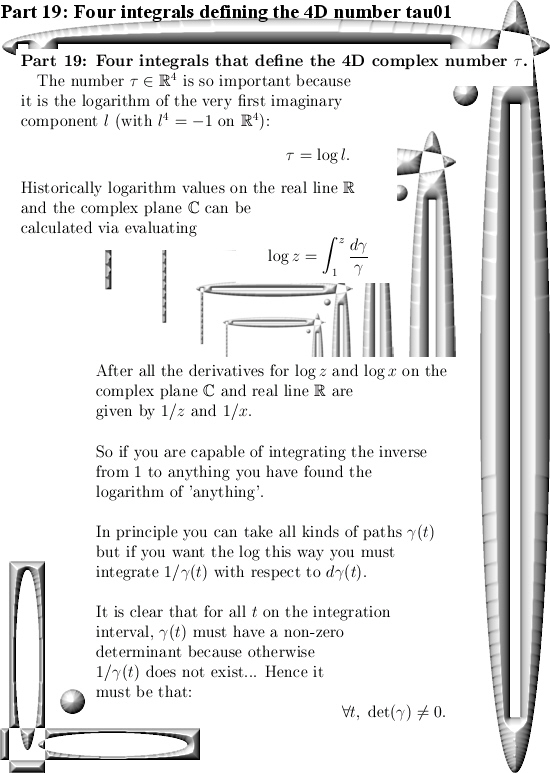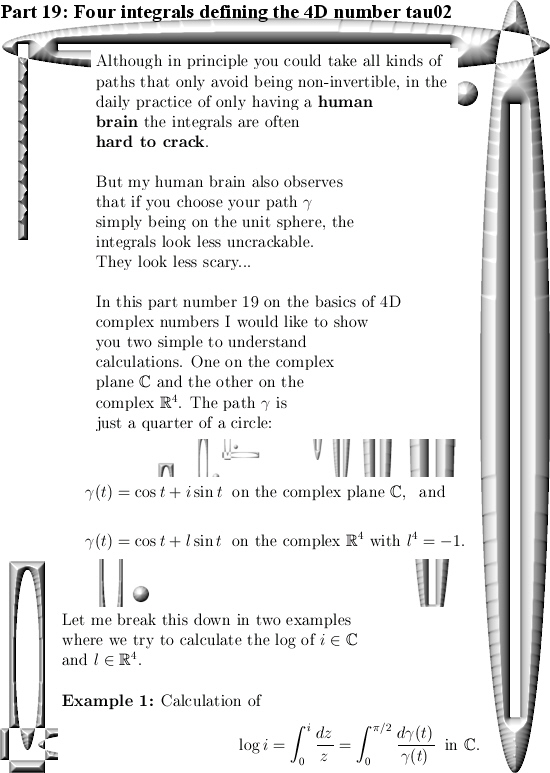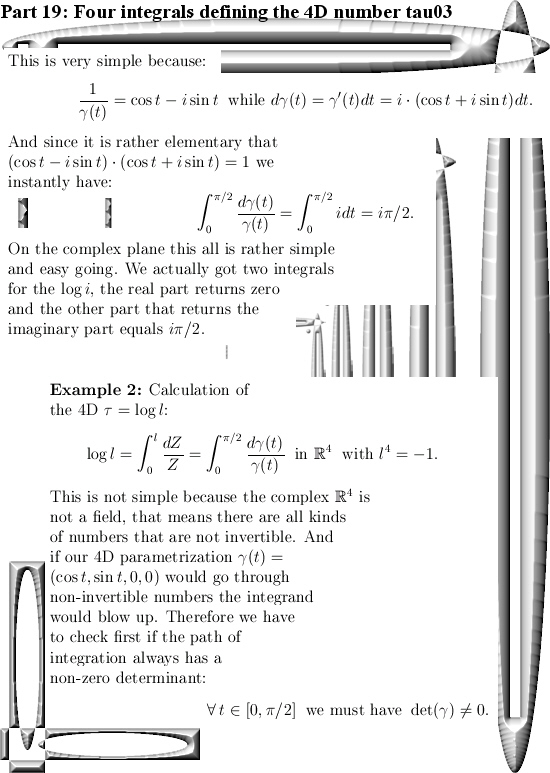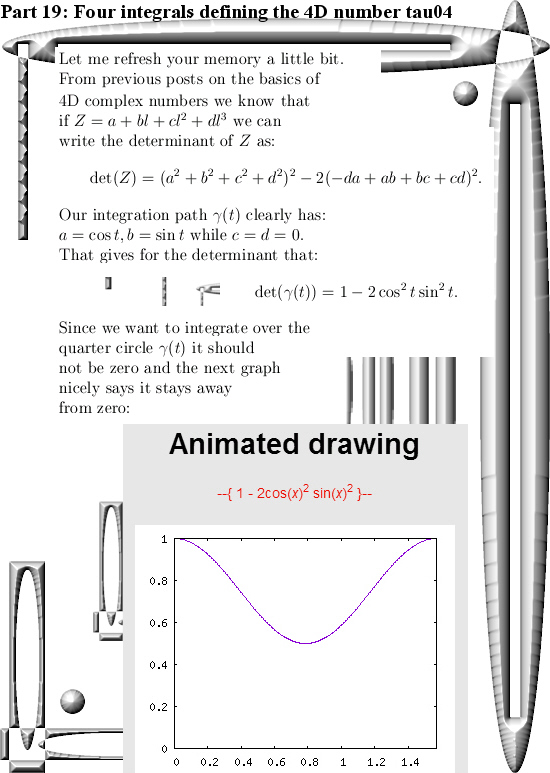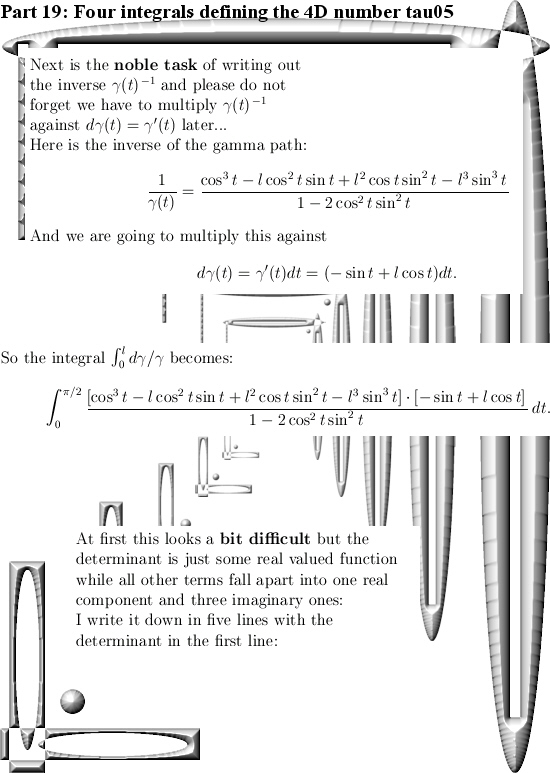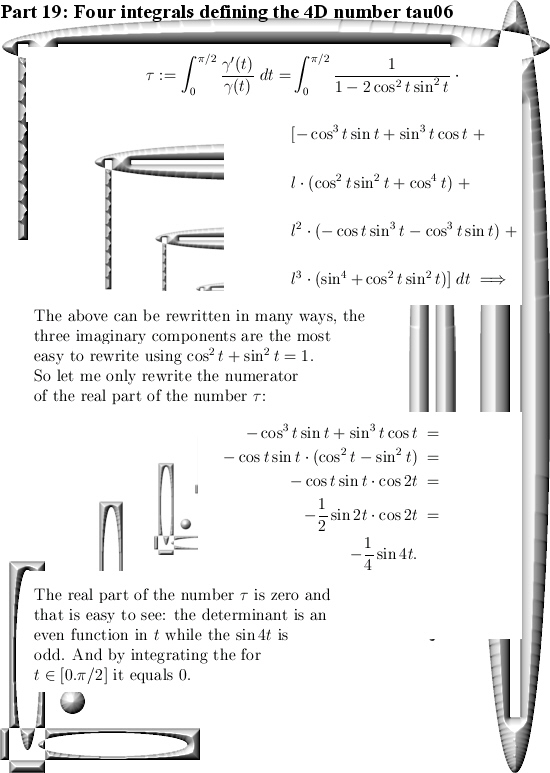End of this post.